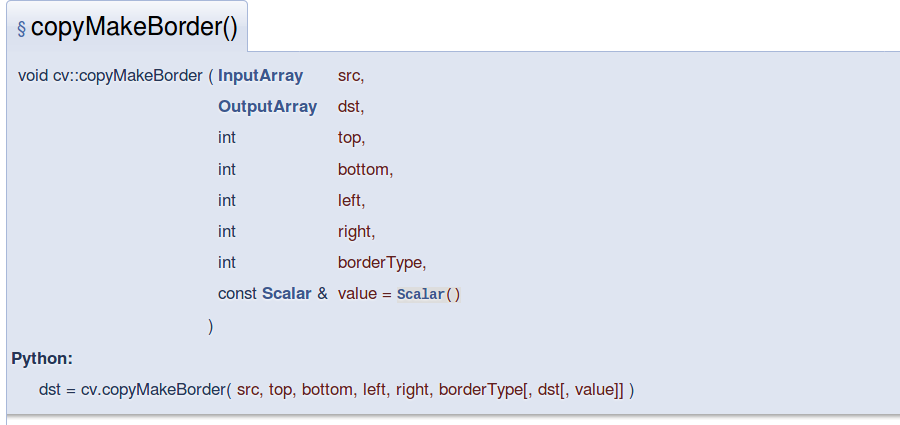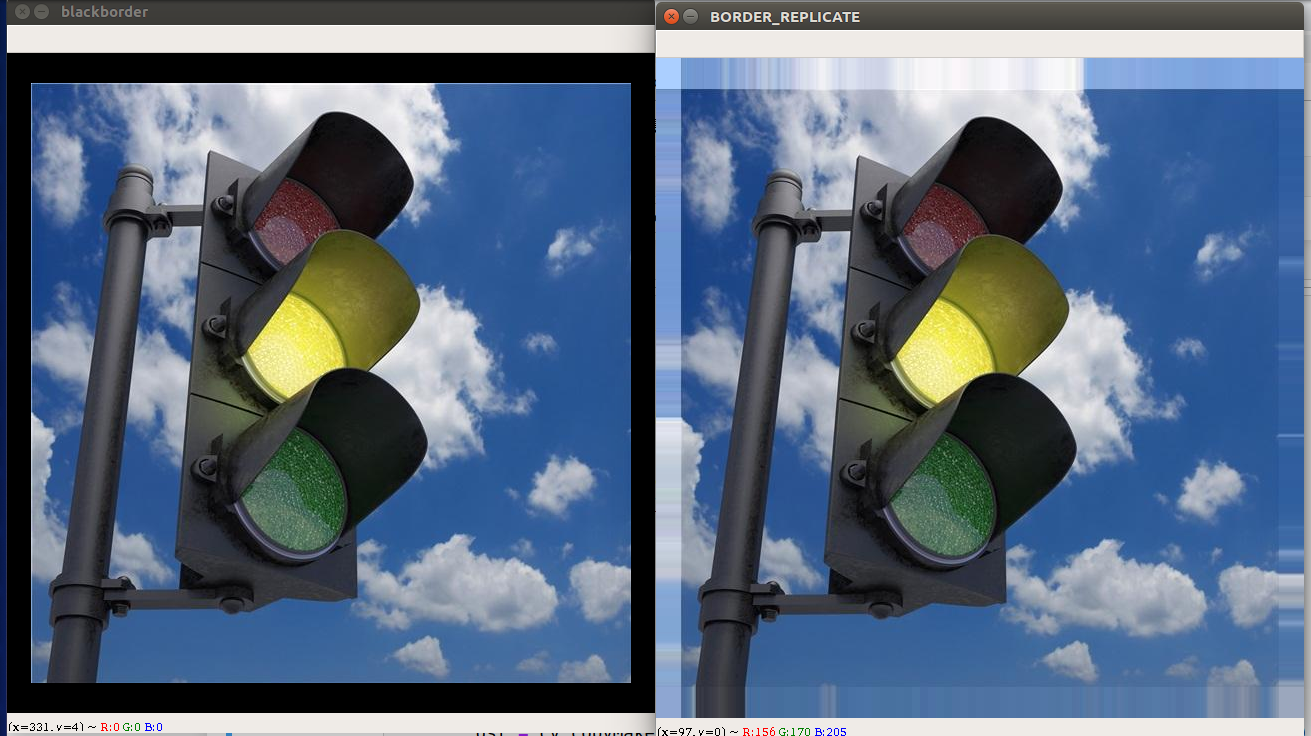Categories

# Add boundary of the image opencv

We often have to do the expansion of demand for image edge. For example,

Hopefully, the same convolution matrix size

I want to change the image size, but does not change the aspect ratio

opencv achieve

opencv中使用copyMakeBorder()来完成这一功能
apisrc is the original image matrix

dst is the new image matrix

top / bottom / left / right boundary is extended size (such as 5 represents 5 pixels)

• borderType
• the pixel value is an extension of what value

borderType in two ways:

BORDER_CONSTANT
Fixed edge padding pixel value, such as black bars filled, 0 is used, white edge 255

BORDER_REPLICATE
Pixel of the original image corresponding edges do filled, the edges of the image seems to be a “pull paste” effect

opencv example

``````import sys
import cv2 as cv

def test():
top = int(0.05 * src.shape)  # shape = rows
bottom = top
left = int(0.04 * src.shape)  # shape = cols
right = left

value = [0,0,0]
borderType = cv.BORDER_CONSTANT
dst1 = cv.copyMakeBorder(src, top, bottom, left, right, borderType, None, value)

borderType = cv.BORDER_REPLICATE
dst2 = cv.copyMakeBorder(src, top, bottom, left, right, borderType, None, value)

cv.imshow("blackborder",dst1)
cv.imshow("BORDER_REPLICATE",dst2)

if 27 == cv.waitKey():
cv.destroyAllWindows()

test()``````